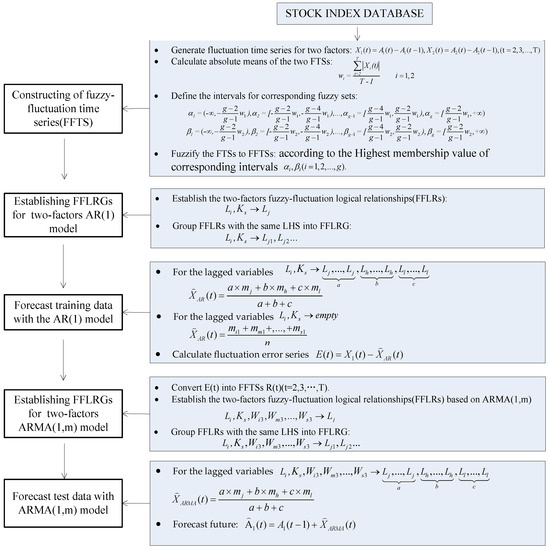Next Article in Journal
Scale Effect and Anisotropy Analyzed for Neutrosophic Numbers of Rock Joint Roughness Coefficient Based on Neutrosophic Statistics
Next Article in Special Issue
A Hybrid Fuzzy DEA/AHP Methodology for Ranking Units in a Fuzzy Environment
Previous Article in Journal
β-Differential of a Graph
Previous Article in Special Issue
A Generalization of Trapezoidal Fuzzy Numbers Based on Modal Interval Theory
Open AccessArticle

A Two-Factor Autoregressive Moving Average Model Based on Fuzzy Fluctuation Logical Relationships

byShuang Guan 1 andAiwu Zhao 2,*
1
Rensselaer Polytechnic Institute, Troy, NY 12180, USA
2
School of management, Jiangsu University, Zhenjiang 212013, China
*
Author to whom correspondence should be addressed.
Symmetry 2017, 9(10), 207; https://doi.org/10.3390/sym9100207
Received: 26 August 2017 / Revised: 25 September 2017 / Accepted: 28 September 2017 / Published: 1 October 2017
(This article belongs to the Special Issue Fuzzy Sets Theory and Its Applications)
Many of the existing autoregressive moving average (ARMA) forecast models are based on one main factor. In this paper, we proposed a new two-factor first-order ARMA forecast model based on fuzzy fluctuation logical relationships of both a main factor and a secondary factor of a historical training time series. Firstly, we generated a fluctuation time series (FTS) for two factors by calculating the difference of each data point with its previous day, then finding the absolute means of the two FTSs. We then constructed a fuzzy fluctuation time series (FFTS) according to the defined linguistic sets. The next step was establishing fuzzy fluctuation logical relation groups (FFLRGs) for a two-factor first-order autoregressive (AR(1)) model and forecasting the training data with the AR(1) model. Then we built FFLRGs for a two-factor first-order autoregressive moving average (ARMA(1,m)) model. Lastly, we forecasted test data with the ARMA(1,m) model. To illustrate the performance of our model, we used real Taiwan Stock Exchange Capitalization Weighted Stock Index (TAIEX) and Dow Jones datasets as a secondary factor to forecast TAIEX. The experiment results indicate that the proposed two-factor fluctuation ARMA method outperformed the one-factor method based on real historic data. The secondary factor may have some effects on the main factor and thereby impact the forecasting results. Using fuzzified fluctuations rather than fuzzified real data could avoid the influence of extreme values in historic data, which performs negatively while forecasting. To verify the accuracy and effectiveness of the model, we also employed our method to forecast the Shanghai Stock Exchange Composite Index (SHSECI) from 2001 to 2015 and the international gold price from 2000 to 2010. View Full-Text
Show FiguresFigure 1

MDPI and ACS Style

Guan, S.; Zhao, A. A Two-Factor Autoregressive Moving Average Model Based on Fuzzy Fluctuation Logical Relationships. Symmetry 2017, 9, 207.

Show more citation formats Show less citations formats
Note that from the first issue of 2016, MDPI journals use article numbers instead of page numbers. See further details here.

1